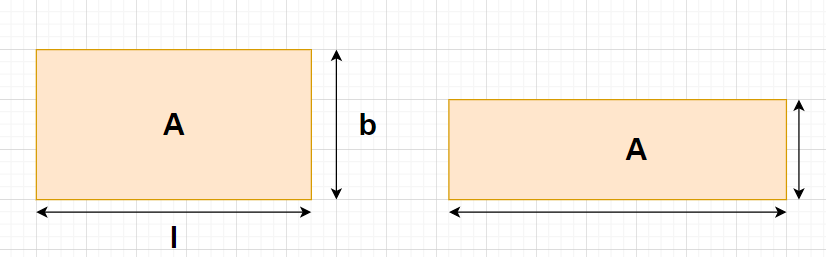QuestionAnswers

# The length of a rectangle is increased by 60 percent. By what percent would the width be decreased so as to maintain the same area?${\text{A}}{\text{. 37}}\dfrac{1}{2}$ percent${\text{B}}{\text{.}}$ 60 percent${\text{C}}{\text{.}}$ 75 percent${\text{D}}{\text{.}}$ None of theseHint- Here, we will proceed by using the formula i.e., the area of any rectangle = (Length of the rectangle)$\times$(Breadth of the rectangle) and then we will come the areas of the rectangles obtained in both the cases given in the problem.

Complete step-by-step solution -
Let us consider a rectangle of length l and breadth b as shown in the figure.
Initial length of the rectangle = l, Initial breadth of the rectangle = b
Since, the area of any rectangle = (Length of the rectangle)$\times$(Breadth of the rectangle) $\to (1)$
Using the formula given by equation (1), we get
Initial area of the rectangle = (Initial length of the rectangle)$\times$(Initial breadth of the rectangle)
$\Rightarrow$Initial area of the rectangle = $l \times b = lb$
It is given that the length of the rectangle is increased by 60 percent
i.e., New length of the rectangle = $\left( {1 + \dfrac{{60}}{{100}}} \right)l = \left( {\dfrac{{100 + 60}}{{100}}} \right)l = \left( {\dfrac{{160}}{{100}}} \right)l = \left( {\dfrac{8}{5}} \right)l$
Let the breadth of the rectangle be decreased by x percent in order to have the same area of the rectangle.
So, New breadth of the rectangle = $\left( {1 - \dfrac{x}{{100}}} \right)b = \left( {\dfrac{{100 - x}}{{100}}} \right)b$
Again using the formula given by equation (1), we get
New area of the rectangle = (New length of the rectangle)$\times$(New breadth of the rectangle)
$\Rightarrow$New area of the rectangle = $\left[ {\left( {\dfrac{8}{5}} \right)l} \right] \times \left[ {\left( {\dfrac{{100 - x}}{{100}}} \right)b} \right] = \left( {\dfrac{8}{5}} \right)\left( {\dfrac{{100 - x}}{{100}}} \right)lb$
Since, it is given that the area obtained in both the cases are equal
i.e., New area of the rectangle = Initial area of the rectangle
$\Rightarrow \left( {\dfrac{8}{5}} \right)\left( {\dfrac{{100 - x}}{{100}}} \right)lb = lb \\ \Rightarrow \left( {\dfrac{8}{5}} \right)\left( {\dfrac{{100 - x}}{{100}}} \right) = 1 \\ \Rightarrow 100 - x = \dfrac{{100 \times 5}}{8} \\ \Rightarrow x = 100 - \dfrac{{125}}{2} \\ \Rightarrow x = \dfrac{{200 - 125}}{2} \\ \Rightarrow x = \dfrac{{75}}{2} \\ \Rightarrow x = 37\dfrac{1}{2} \\$
Therefore, the width of the rectangle needs to be decreased by $37\dfrac{1}{2}$ percent so as to maintain the same area.
Hence, option A is correct.

Note- In this particular problem, the area of the rectangle needs to be the same i.e., the product of the length and breadth of the rectangle needs to be the same. Since, the length of the rectangle is increasing so the breadth of the rectangle must be decreasing in order to have a constant area of the rectangle.